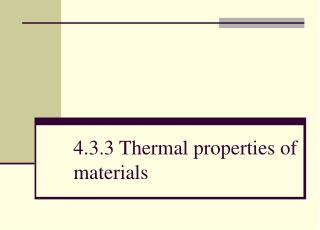DownloadDownload Presentation4.3.3 Thermal properties of materials

# 4.3.3 Thermal properties of materials

Download Presentation## 4.3.3 Thermal properties of materials

- - - - - - - - - - - - - - - - - - - - - - - - - - - E N D - - - - - - - - - - - - - - - - - - - - - - - - - - -
##### Presentation Transcript

1. 4.3.3 Thermal properties of materials

2. Objective (a) define and apply the concept of specific heat capacity

3. Objective (b) select and apply the equation E = mcΔθ

4. Specific Heat Capacity If we heat matter so that its temperature rises, the amount of energy we must supply depends on three things: • The mass m of the material • The temperature rise Δθ we wish to achieve • The material itself

5. Specific Heat Capacity ΔQ = mcΔθ where ΔQ = energy supplied (J) m = mass (kg) c = specific heat capacity (J kg-1 K-1) Δθ = change in temperature (°C or K)

6. Specific Heat Capacity The specific heat capacity of a substance is numerically equal to the amount of energy required to raise the temperature of 1kg of the substance by 1 K (or by 1 °C)

7. Specific Heat Capacity When 26 400 J of energy is supplied to a 2kg block of aluminium, its temperature rises from 20 °C to 35 °C. Find the specific heat capacity of aluminium. c = ΔQ / mΔθ c = 26 400 J / (2 kg x 15 K) c = 880 J kg-1 K-1

8. Specific Heat Capacity • How much energy must be supplied to raise the temperature of 5 kg of water from 20°C to 100°C? • Which requires more energy, heating a 2 kg block of lead by 30 K, or heating a 4 kg block of copper by 5 K? • A well-insulated 1 kg block of iron is heated using a 50 W heater for 5 min. Its temperature rises from 22°C to 55°C. Find the specific heat capacity of iron.

9. Objective c) describe an electrical experiment to determine the specific heat capacity of a solid or liquid

10. SHC Practical 12 V • Run for 1200 seconds • Take temperature every 30 s • Draw graph • Calculate c • Repeat for different substance heater thermometer metal block

11. SHC Practical • calculate gradient Δθ Δt • ΔQ = mc Δθ • VIΔt = mc Δθ • c = VI Δt m Δθ • c = VI 1 m Δt Δθ temp (°C) time (s)

12. SHC Practical

13. Objective d) describe what is meant by the terms latent heat of fusion and latent heat of vaporisation

14. Specific Latent Heat What is happening at: • AB? • CD? E C 100 D θ • AB: Melting – particles becoming disordered • CD: Boiling – particles completely separating A 0 B O t

15. Specific Latent Heat • At AB and CD, energy is being input, but the temperature isn’t rising • The energy is being used to break the molecules free, not raise the temperature

16. Specific Latent Heat • At AB (melting) and CD (boiling) • energy input • temperature does not change • molecules become disordered (AB) or separate from each other (CD) • little change in kinetic energy • electrical potential energy increases

17. Specific Latent Heat • At OA and BC and DE • energy input • temperature rises • molecules move faster • kinetic energy increases (temperature = average ke) • little change in electrical potential energy

18. Specific Latent Heat • The energy needed to cause this change of state is Latent Heat (“Latent” means “hidden”) • When a substance melts, this is the latent heat of fusion • When a substance boils, this is the latent heat of vaporisation

19. Definitions • Latent heat of fusion is the energy which must be supplied to cause a substance to melt at a constant temperature • Latent heat of vaporisation is the energy which must be supplied to cause a substance to boil at a constant temperature

20. Specific Latent Heat Remember: • Temperature is a measure of the average kinetic energy of the molecules • When a thermometer is put into water, the water molecules collide with the thermometer and share their kinetic energy with it. • At a change of state, there is no change in kinetic energy, so no change of temperature

21. Specific Latent Heat Why does it take more energy to boil a substance than it does to melt it? Melting – molecules still bonded to most of their neighbours – breaks one or two bonds Boiling – each molecule breaks free from all of its neighbours – breaks eight or nine bonds

22. Specific Latent Heat The specific latent heat of a substance is the energy required per kilogram of the substance to change its state without any change of temperature

23. Specific Latent Heat The specific latent heat of vaporisation of water is 2.26 MJ kg-1. Calculate the energy needed to change 2.0 g of water into steam at 100 °C. • 1.0 kg (1000 g) of water requires 2.26 MJ of energy • Therefore, energy = 2.0/1000 x 2.26 x 106 = 4520 J

24. Specific Latent Heat The specific latent heat of fusion of water is 330 kJ kg-1. Calculate the energy needed to change 2.0 g of ice into water at 0 °C. • 1.0 kg (1000 g) of ice requires 330 kJ of energy • Therefore, energy = 2.0/1000 x 3.30 x 105 = 660 J

25. Practical